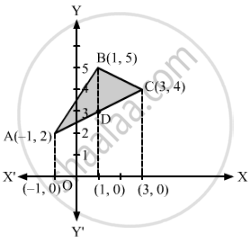# Using integration, find the area of the region bounded by the triangle whose vertices are (−1, 2), (1, 5) and (3, 4). - Mathematics

Using integration, find the area of the region bounded by the triangle whose vertices are (−1, 2), (1, 5) and (3, 4).

#### Solution

Let ABC be the required triangle with vertices A (−1, 2), B (1, 5) and C (3, 4).

Now, the equation of the side AB is

y−2=(5−2)/(1−(−1))(x−(−1))

⇒ 3x − 2y + 7 = 0             ... (i)

The equation of BC is

y−5=(4−5)/(3−1)(x−1)

⇒ x + 2y − 11 = 0             ... (ii)

The equation of AC is

y−2=(4−2)/(3−(−1))(x−(−1))

⇒ x − 2y + 5 = 0             ... (iii)Now, area of ΔABC = ∫_(−1)^1yABdx+∫_1^3yBCdx−∫_(−1)^3yACdx

=∫_(-1)^1(3x+7)/2dx + ∫_1^3(11−x)/2dx − ∫_1^3(x+5)/2dx

=12[((3x^2)/2+7x)_(-1)^1+(11x−x^2/2)_1^3−(x^2/2+5x)_(-1)^3]

=1/2[(3/2+7−3/2+7)+(33−9/2−11+12)−(9/2+15−1/2+5)]

= 1/2 × 8 = 4 sq. units

Concept: Area Between Two Curves
Is there an error in this question or solution?